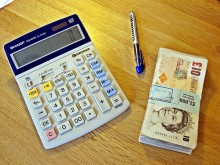# How Do I Calculate A Percentage On A Calculator?To calculate percentage on a calculator, first find 1% of the number, and multiply this by the percentage that you are trying to find.  Eg: To find 40% of 200; Divide 200 by 100 = 2 and multiply this by 40 = 80

thanked the writer.
6000*20%
thanked the writer.
For instance ,to find say 35 percent of 70 ,you would enter 70 then the multiply key then enter 35 and then percent key giving the answer which is 24.5 %
thanked the writer.
Anonymous commented
Wrong. For instance, to fine say 35% of 70, you would enter 70 then DIVIDE key then enter 35 and then the % key giving the answer which is 24.5%
I need to find out 2.1 million is what percentage of 8.6 million?
thanked the writer.
Example. 10 X 10% the 10% converts to .10 you always move the point two places to the left of the last number. So 10% of 10 is 1. If you need further explanation just ask me, or give me the problem.
thanked the writer.
There was 20 problems and I made 47% whats my grade
thanked the writer.
6000*20%
thanked the writer.
The answer is \$1200.00 .Enter 6000 and then the multiply key and then enter 20 and the percent key.
thanked the writer.
Enter the number than press the multiplication sign then the percent you want to get then hit =..
thanked the writer.
Percent = hundredths
Therefore:
20% = 20/100 = 0.20
0.2*6000 = 20% of 6000

What you call 0.2 is a change factor.
thanked the writer.
Simply multiply or divide the number by the "other" number to determine whether or not your desired answered is of the percentage of or the number is that what percentage. E.g. What is 5% of 20? =1 opposed to what is the percentage of 1 that equals 20? = 5%
thanked the writer.